# extractLBPFeatures

Extract local binary pattern (LBP) features

## Syntax

``features = extractLBPFeatures(I)``
``features = extractLBPFeatures(I,Name,Value)``

## Description

````features = extractLBPFeatures(I)` returns extracted uniform local binary pattern (LBP) from a grayscale image. The LBP features encode local texture information.```

example

````features = extractLBPFeatures(I,Name,Value)` uses additional options specified by one or more `Name,Value` pair arguments.```

## Examples

collapse all

Read images that contain different textures.

```brickWall = imread('bricks.jpg'); rotatedBrickWall = imread('bricksRotated.jpg'); carpet = imread('carpet.jpg');```

Display the images.

```figure imshow(brickWall) title('Bricks')```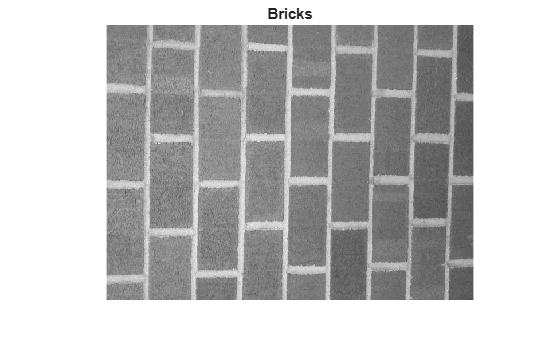```figure imshow(rotatedBrickWall) title('Rotated Bricks')```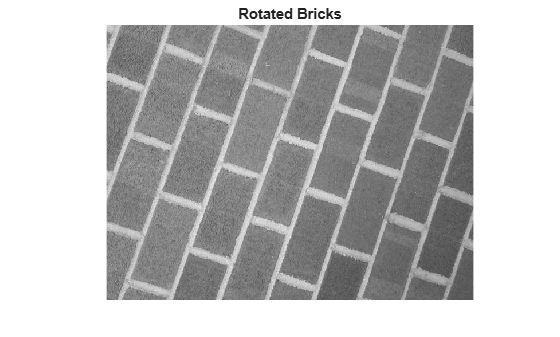```figure imshow(carpet) title('Carpet')```Extract LBP features from the images to encode their texture information.

```lbpBricks1 = extractLBPFeatures(brickWall,'Upright',false); lbpBricks2 = extractLBPFeatures(rotatedBrickWall,'Upright',false); lbpCarpet = extractLBPFeatures(carpet,'Upright',false);```

Gauge the similarity between the LBP features by computing the squared error between them.

```brickVsBrick = (lbpBricks1 - lbpBricks2).^2; brickVsCarpet = (lbpBricks1 - lbpCarpet).^2;```

Visualize the squared error to compare bricks versus bricks and bricks versus carpet. The squared error is smaller when images have similar texture.

```figure bar([brickVsBrick; brickVsCarpet]','grouped') title('Squared Error of LBP Histograms') xlabel('LBP Histogram Bins') legend('Bricks vs Rotated Bricks','Bricks vs Carpet')```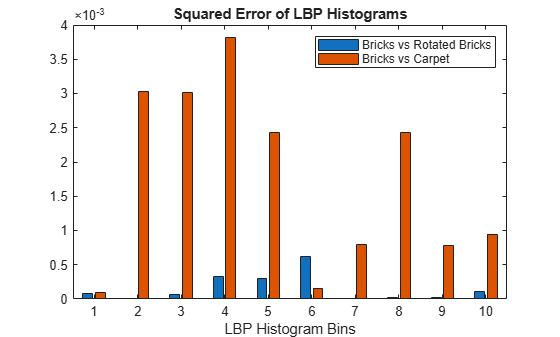Read in a sample image and convert it to grayscale.

```I = imread('gantrycrane.png'); I = rgb2gray(I);```

Extract unnormalized LBP features so that you can apply a custom normalization.

`lbpFeatures = extractLBPFeatures(I,'CellSize',[32 32],'Normalization','None');`

Reshape the LBP features into a number of neighbors -by- number of cells array to access histograms for each individual cell.

```numNeighbors = 8; numBins = numNeighbors*(numNeighbors-1)+3; lbpCellHists = reshape(lbpFeatures,numBins,[]);```

Normalize each LBP cell histogram using L1 norm.

`lbpCellHists = bsxfun(@rdivide,lbpCellHists,sum(lbpCellHists));`

Reshape the LBP features vector back to 1-by- N feature vector.

`lbpFeatures = reshape(lbpCellHists,1,[]);`

## Input Arguments

collapse all

Input image, specified as an M-by-N 2-D grayscale image that is real, and non-sparse.

Data Types: `logical` | `single` | `double` | `int16` | `uint8` | `uint16`

### Name-Value Pair Arguments

Specify optional comma-separated pairs of `Name,Value` arguments. `Name` is the argument name and `Value` is the corresponding value. `Name` must appear inside quotes. You can specify several name and value pair arguments in any order as `Name1,Value1,...,NameN,ValueN`.

Example: `'NumNeighbors'`,`8`

Algorithm Parameters
The LBP algorithm parameters control how local binary patterns are computed for each pixel in the input image.

Number of neighbors used to compute the LBP for each pixel in the input image, specified as the comma-separated pair consisting of '`NumNeighbors`' and a positive integer. The set of neighbors is selected from a circularly symmetric pattern around each pixel. Increase the number of neighbors to encode greater detail around each pixel. Typical values range from `4` to `24`.

Radius of circular pattern used to select neighbors for each pixel in the input image, specified as the comma-separated pair consisting of '`Radius`' and a positive integer. To capture detail over a larger spatial scale, increase the radius. Typical values range from `1` to `5`.

Rotation invariance flag, specified as the comma-separated pair consisting of '`Upright`' and a logical scalar. When you set this property to `true`, the LBP features do not encode rotation information. Set '`Upright`' to `false` when rotationally invariant features are required.

Interpolation method used to compute pixel neighbors, specified as the comma-separated pair consisting of '`Interpolation`' and either `'Linear'` or `'Nearest'`. Use `'Nearest'` for faster computation, but with less accuracy.

Histogram Parameters
The histogram parameters determine how the distribution of binary patterns is aggregated over the image to produce the output features.

Cell size, specified as the comma-separated pair consisting of '`CellSize`' and a 2-element vector. The number of cells is calculated as `floor`(`size`(`I`)/`CellSize`).

Type of normalization applied to each LBP cell histogram, specified as the comma-separated pair consisting of '`Normalization`' and either `'L2'` or `'None'`. To apply a custom normalization method as a post-processing step, set this value to `'None'`.

## Output Arguments

collapse all

LBP feature vector, returned as a 1-by-N vector of length N representing the number of features. LBP features encode local texture information, which you can use for tasks such as classification, detection, and recognition. The function partitions the input image into non-overlapping cells. To collect information over larger regions, select larger cell sizes . However, when you increase the cell size, you lose local detail. N, depends on the number of cells in the image, numCells, the number of neighbors, P, and the `Upright` parameter.

The number of cells is calculated as:

 numCells = prod(`floor`(`size`(`I`)/`CellSize`))

The figure shows an image with nine cell histograms. Each histogram describes an LBP feature.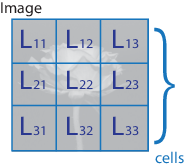The size of the histogram in each cell is [1,B], where B is the number of bins in the histogram. The number of bins depends on the `Upright` property and the number of neighbors, P.

Upright Number of Bins
`true`(P x P–1) + 3)
`false`(P + 2)

The overall LBP feature length, N, depends on the number of cells and the number of bins, B:

 N = numCells x B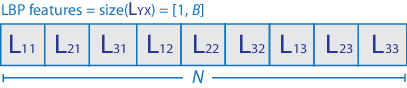Ojala, T., M. Pietikainen, and T. Maenpaa. “Multiresolution Gray Scale and Rotation Invariant Texture Classification With Local Binary Patterns.” IEEE Transactions on Pattern Analysis and Machine Intelligence. Vol. 24, Issue 7, July 2002, pp. 971-987.